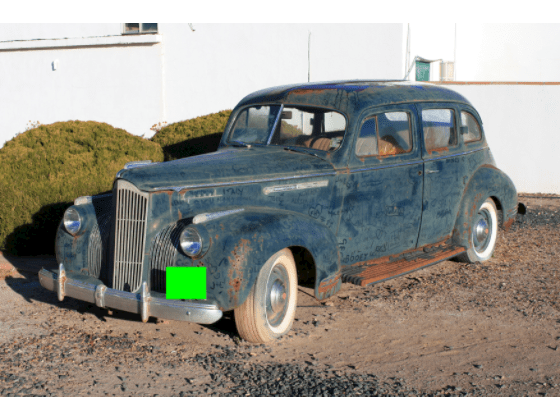Main Content

# imerase

Remove image pixels within rectangular region of interest

## Syntax

``Ierased = imerase(I,rect)``
``Ierased = imerase(I,rect,'FillValues',fillValues)``

## Description

example

````Ierased = imerase(I,rect)` remove pixels of image `I` within the rectangular region defined by `rect` and returns the image with the erased region, `Ierased`.```

example

````Ierased = imerase(I,rect,'FillValues',fillValues)` also specifies the fill value to apply to the erased pixels.```

## Examples

collapse all

Read and display an image.

```I = imread("peppers.png"); imshow(I)```Select a rectangular region of size 50-by-100 pixels from a random location in the image.

`rect = randomWindow2d(size(I),[50 100]);`

Erase the pixels within the rectangular region.

`J = imerase(I,rect);`

Display the erased image. The erased pixels have the value `0` and appear black.

`imshow(J)`Read and display an image.

```I = imread("car1.jpg"); imshow(I)```Specify the size and position of the erase rectangle as a 4-element vector of the form [xmin ymin width height].

`rect = [1040 1525 250 200];`

Erase the pixels from within the rectangular region, and fill the erased pixels with the color green.

`J = imerase(I,rect,"FillValues",[0 255 0]);`

Display the erased image.

`imshow(J)`Read and display a color image.

```I = imread('flamingos.jpg'); imshow(I)```Select a random square window from the image. The area of the window is between 2% and 13% of the area of the entire image.

`win = randomWindow2d(size(I),"Scale",[0.02 0.13],"DimensionRatio",[1 1;1 1]);`

Determine the height and width of the erase region.

```hwin = diff(win.YLimits)+1; wwin = diff(win.XLimits)+1;```

Erase the pixels within the erase region. Fill each pixel with a random color.

`J = imerase(I,win,"FillValues",randi([1 255],[hwin wwin 3]));`

Display the erased image.

`imshow(J)`## Input Arguments

collapse all

Image with a region to be erased, specified as a numeric matrix representing a grayscale image or a numeric array with three channels representing a color image.

Size and position of the erase rectangle, specified as a 4-element numeric vector of the form [xmin ymin width height] or an `images.spatialref.Rectangle` object.

Fill value to apply to erased pixels, specified as one of these values.

Fill ValueResult
numeric scalarFill erased pixels of a grayscale or RGB image with the specified gray value.
3-element numeric vectorFill erased pixels of an RGB image with the specified color.
numeric matrixFill each erased pixel of a grayscale or RGB image with the corresponding gray value in `fillValue`. The matrix specified by `fillValue` must have the same height and width as the erase rectangle, `rect`.
numeric array with 3 planesFill each erased pixel of an RGB image with the color in the corresponding pixel of `fillValue`. The array specified by `fillValue` must have the same height and width as the erase rectangle, `rect`.

## Output Arguments

collapse all

Image with erased region, returned as a numeric matrix or numeric array of the same size as the input image, `I`.

## See Also

Introduced in R2021a